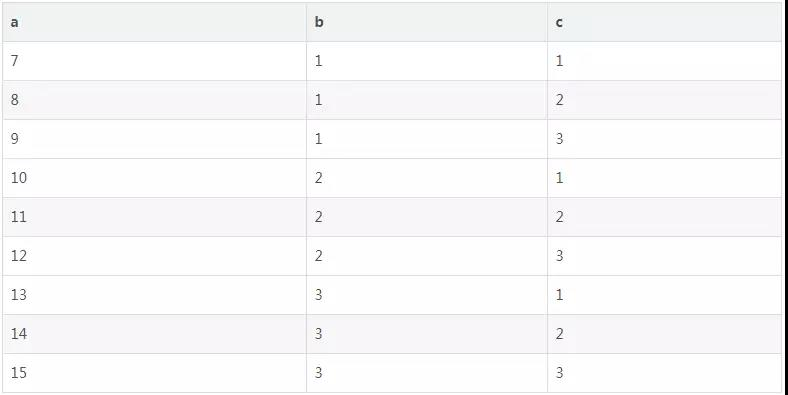2019
07-06

# MySQL 如何查找删除重复行？```create table test(id int not null primary key, day date not null);   insert into test(id, day) values(1, '2006-10-08');   insert into test(id, day) values(2, '2006-10-08');   insert into test(id, day) values(3, '2006-10-09');   select * from test;
+----+------------+   | id | day        |
+----+------------+   |  1 | 2006-10-08 |
|  2 | 2006-10-08 |
|  3 | 2006-10-09 |
+----+------------+ ```

```select day, count(*) from test GROUP BY day;
+------------+----------+   | day        | count(*) |
+------------+----------+
| 2006-10-08 |        2 |   | 2006-10-09 |        1 |
+------------+----------+ ```

```select day, count(*) from test group by day HAVING count(*) > 1;
+------------+----------+
| day        | count(*) |
+------------+----------+
| 2006-10-08 |        2 |
+------------+----------+```

```create temporary table to_delete (day date not null, min_id int not null);   insert into to_delete(day, min_id)
select day, MIN(id) from test group by day having count(*) > 1;   select * from to_delete;
+------------+--------+   | day        | min_id |
+------------+--------+   | 2006-10-08 |      1 |
+------------+--------+ ```

```delete from test
where exists(
select * from to_delete
where to_delete.day = test.day and to_delete.min_id <> test.id
)```

```create table a_b_c(
a int not null primary key auto_increment,
b int,
c int
);   insert into a_b_c(b,c) values (1, 1);   insert into a_b_c(b,c) values (1, 2);   insert into a_b_c(b,c) values (1, 3);   insert into a_b_c(b,c) values (2, 1);   insert into a_b_c(b,c) values (2, 2);   insert into a_b_c(b,c) values (2, 3);   insert into a_b_c(b,c) values (3, 1);   insert into a_b_c(b,c) values (3, 2);   insert into a_b_c(b,c) values (3, 3);```

```select b, c, count(*) from a_b_c   group by b, c   having count(distinct b > 1)
or count(distinct c > 1);```

```select b, c, count(*) from a_b_c   group by b, c   having count(1)
or count(1);```

```select b, c, count(*) from a_b_c   group by b, c   having count(distinct b) > 1
or count(distinct c) > 1;```

```select b, count(*) from a_b_c group by b having count(distinct c) > 1;
+------+----------+   | b    | count(*) |
+------+----------+
|    1 |        3 |   |    2 |        3 |
|    3 |        3 |
+------+----------+``````select b as value, count(*) as cnt, 'b' as what_col   from a_b_c group by b having count(*) > 1   union   select c as value, count(*) as cnt, 'c' as what_col   from a_b_c group by c having count(*) > 1;
+-------+-----+----------+   | value | cnt | what_col |
+-------+-----+----------+   |     1 |   3 | b        |
|     2 |   3 | b        |
|     3 |   3 | b        |
|     1 |   3 | c        |
|     2 |   3 | c        |
|     3 |   3 | c        |
+-------+-----+----------+ ```

```select a, b, c from a_b_c
where b in (select b from a_b_c group by b having count(*) > 1)
or c in (select c from a_b_c group by c having count(*) > 1);
+----+------+------+   | a  | b    | c    |
+----+------+------+   |  7 |    1 |    1 |   |  8 |    1 |    2 |   |  9 |    1 |    3 |   | 10 |    2 |    1 |   | 11 |    2 |    2 |   | 12 |    2 |    3 |   | 13 |    3 |    1 |   | 14 |    3 |    2 |   | 15 |    3 |    3 |
+----+------+------+```

```select a, a_b_c.b, a_b_c.c   from a_b_c
left outer join (
select b from a_b_c group by b having count(*) > 1
) as b on a_b_c.b = b.b
left outer join (
select c from a_b_c group by c having count(*) > 1
) as c on a_b_c.c = c.c   where b.b is not null or c.c is not null ```